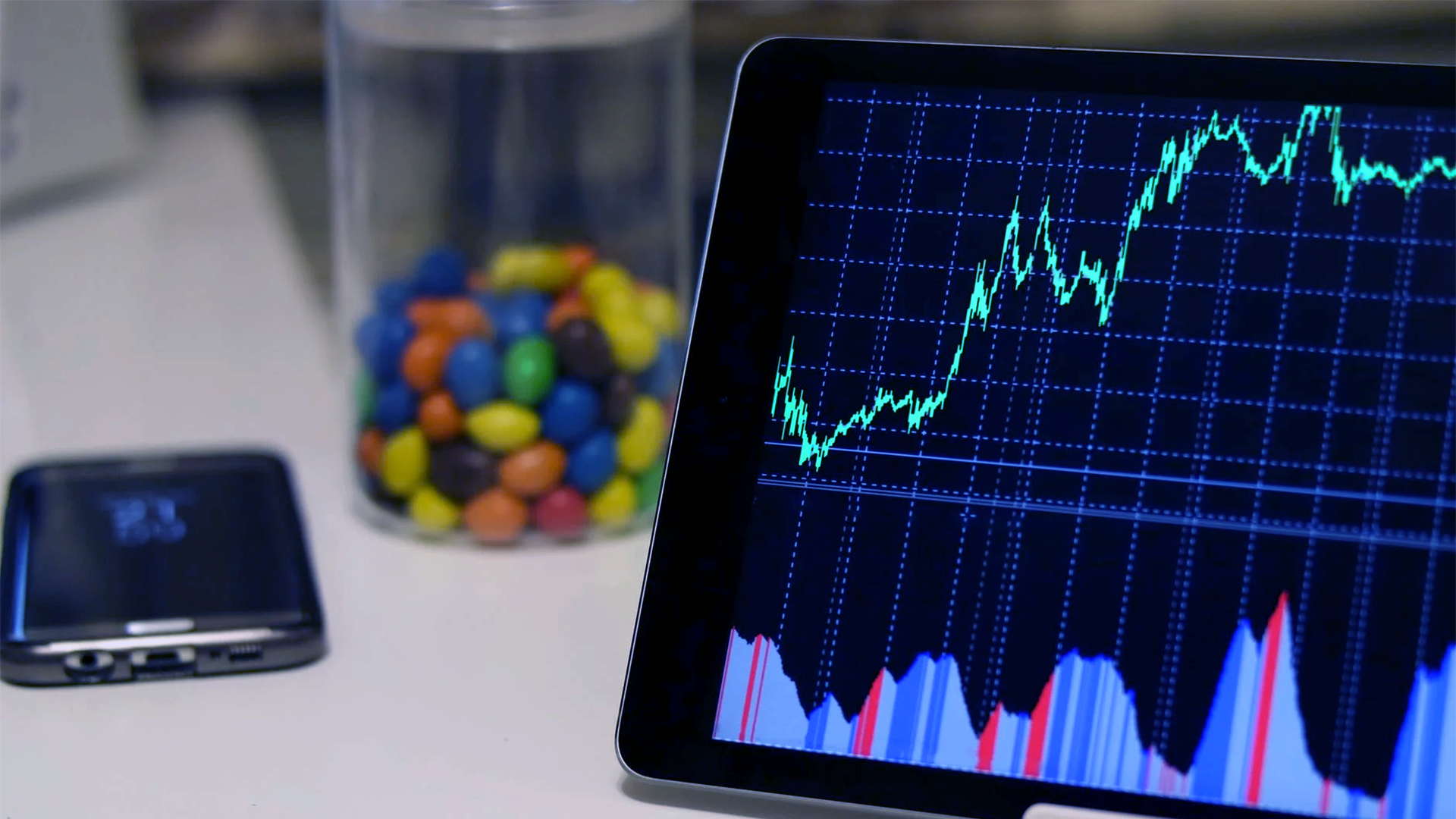# Monte Carlo Simulation An In-depth Tutorial with Python

Original article was published by Towards AI Team on Artificial Intelligence on Medium# 4. Buffon’s Needle Problem:

A French nobleman Georges-Louis Leclerc, Comte de Buffon, posted the following problem in 1777  .

Suppose that we drop a short needle on a ruled paper — what would be the probability that the needle comes to lie in a position where it crosses one of the lines?

The probability depends on the distance (d) between the lines of the ruled paper, and it depends on the length (l) of the needle that we drop — or rather, it depends on the ratio `l/d`. For this example, we can interpret the needle as `l ≤ d`. In short, our purpose is that the needle cannot cross two different lines at the same time. Surprisingly, the answer to the Buffon’s needle problem involves PI.

Here we are going to use the solution of Buffon’s needle problem to estimate the value of PI experimentally using the Monte Carlo Method. However, before going into that, we are going to show how the solution derives, making it more interesting.

## Theorem:

If a short needle, of length l, is dropped on a paper that is ruled with equally spaced lines of distance d ≥ l, then the probability that the needle comes to lie in a position where it crosses one of the lines is:

## Proof:

Next, we need to count the number of needles that crosses any of the vertical lines. For a needle to intersect with one of the lines, for a specific value of theta, the following are the maximum and minimum possible values for which a needle can intersect with a vertical line.

1. Maximum Possible Value:

2. Minimum Possible Value:

Therefore, for a specific value of theta, the probability for a needle to lie on a vertical line is:

The above probability formula is only limited to one value of theta; in our experiment, the value of theta ranges from 0 to pi/2. Next, we are going to find the actual probability by integrating it concerning all the values of theta.

## Estimating PI using Buffon’s needle problem:

Next, we are going to use the above formula to find out the value of PI experimentally.

Now, notice that we have the values for l and d. Our goal is to find the value of P first so that we can get the value of PI. To find the probability P, we must need the count of hit needles and total needles. Since we already have the count of total needles, the only thing we require now is the count of hit needles.

Below is the visual representation of how we are going to calculate the count of hit needles.

## Python Implementation:

1. Import required libraries:

2. Main function:

3. Calling the main function:

4. Output:

As shown in figure 37, after 100 iterations we are able to get a very close value of PI using the Monte Carlo Method.